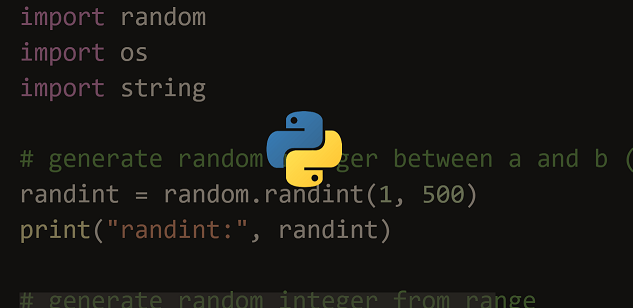# How to Randomly Select Item from List in Python

Sometimes you may need to randomly select one or more items from list in python. You can easily do this using random module in python. It is a built-in module in python and does not require any installation. In this article, we will learn how to randomly select item from list in python.

## How to Randomly Select Item from List in Python

Here are the steps to randomly select items from python list. Let us say you have the following list.

`a=[1,2,3,4,5]`

We will use random.choice() function to select one item from python list. Its syntax is as follows.

`random.choice(list)`

You need to specify the list from which the random item to be returned, in random.choice() function.

Here is the code to select random item from this list.

```import random
print(random.choice(a))
3```

In the above code, random.choice() function will automatically return a random item from the list. Please note, if you call this function immediately again, it may return a different element.

```import random
print(random.choice(a))
1
print(random.choice(a))
5```

So if you wish to re-use this random value in your code, it is advisable to assign it to a variable first, before re-using it.

```import random
b=random.choice(a)
print b
2
print b
2```

## How to Select Multiple Random Items from List

Sometimes you may need to select multiple items from a given python list. In such cases, we will use random.sample() function. Its syntax is as follows.

`random.sample(list,no_of_elements)`

In the above code, you need to specify the list and the number of random elements to be returned. Here is an example to return 2 random elements from python list.

```import random
random.sample(a,2)
[5,3]```

Please note, the order of items may be different in your result, compared to the original result. In some python versions. random.sample() function may not be available. In such cases, use random.choices() function which has the same syntax.

```import random
random.choices(a,2)
[5,3]```

Please note, both the above functions require a list as the first argument. If you want to select random item from other data structures such as tuples or dictionaries, then you will need to convert them into list and then pass them to the above functions.

In this article, we have learnt how to select random items from list. You can customize the above functions as per your requirement.